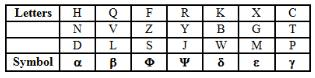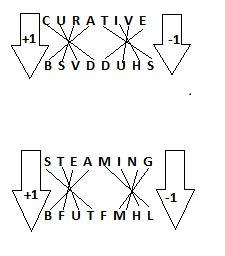Courses

# Coding - Decoding MCQ 2

## 20 Questions MCQ Test IBPS PO Mains - Study Material, Online Tests, Previous Year | Coding - Decoding MCQ 2

Description
This mock test of Coding - Decoding MCQ 2 for SSC helps you for every SSC entrance exam. This contains 20 Multiple Choice Questions for SSC Coding - Decoding MCQ 2 (mcq) to study with solutions a complete question bank. The solved questions answers in this Coding - Decoding MCQ 2 quiz give you a good mix of easy questions and tough questions. SSC students definitely take this Coding - Decoding MCQ 2 exercise for a better result in the exam. You can find other Coding - Decoding MCQ 2 extra questions, long questions & short questions for SSC on EduRev as well by searching above.
QUESTION: 1

### If REUNION LIMITED is written as QAOMEIM KELESAC, how will SCHOOL be written?

Solution:

C is the correct option.In the given coded word each constant is substituted by preceding constant and each vowel is also substituted by preceding vowel. Hence, SCHOOL will be codes as RBGIIK.

QUESTION: 2

### If ROME BOMBAY is written as SUNI CUNCEZ, how will ASIA will be written?

Solution:

Vowel is coded by the next vowel and consonant is coded by the next consonant. Therefore, using the same pattern ASIA will be coded as ETOE.

QUESTION: 3

### If TEMPORARY is written as YRAROPMET, how will PERMANENT will be written?

Solution:
QUESTION: 4

If TEMPORARY is written as GVNKLIZIB how will PERMANENT be written?

Solution:

The correct option is B

The formula is = 27 - no. of the alphabet on which it is placed. = the answer

27 - 20(T) = 7(G)

Similarly the whole word will be done like this

Also

27 - (P)16 = 11 (K)

27 - (E)5  = 22(V)

And so on...

QUESTION: 5

In a certain code language, 123 means ‘I prefer coffee’, 356 means ‘They dislike coffee’, and 589 means ‘They want tea’, which digit stands for ‘dislike’?

Solution:

In the first and second statements, the common code digit is '3' and the common word is 'coffee'.
So, '3' means 'coffee'.
In the second and third statements, the common code digit '5' and the common word is 'they'.
So, '5' means 'they'.
Thus, in the second statements, '6' means 'dislike'.

QUESTION: 6

If in a certain language BLEMISH is coded as AODPHVG, how is CHAPTER coded in that code?

Solution:
QUESTION: 7

In a certain code “ Nee Chi Tee” means “Ring the bell”, “Ki chi Ba” means “Bell is black” and “Su nee ki la” means “The pen is red. Which code stands for ‘Ring’?

Solution:
QUESTION: 8

If man is called lady, lady is called girl. Girl is called boy, boy is called butler and butler is called rogue, who will serve in a restaurant.

Solution:
QUESTION: 9

If in a certain language 943 is coded as BED and 12448 is coded as SWEET. How is 492311 coded in that languages?

Solution:
QUESTION: 10

If TEMPORARY is written as BEDGFIAIG, how will PERMANENT be written?

Solution:
QUESTION: 11

In a certain code language BLUNDER is written as LBNURED. Then how will QUICKER be written in that code?

Solution:
QUESTION: 12

In a certain code language

I. ‘Do No Pa’ means ‘Amit is lucky’.

II.  ‘Po Cho To’ means ‘She was there’.

III. Po No Bo’ means ‘She is beautiful’.

Then which of the following is the code for ‘lucky’?

Solution:
QUESTION: 13

How will you write ‘is’ in a certain code?

1. Old is Gold means ‘cha ta ni’
2. Gold to Sold means ‘si ni ki’
3. me fold old means ‘ti pa ta’
Solution: Gold = ni

old = ta

is = cha

So option C
QUESTION: 14

In a certain code language the word FUNCTION is written as UCINHPVQ. How will the word REGULATE be written in that language?

Solution:https://edurev.in/allimages/original/applicationimages/temp/59_e0532418-99f9-4dac-b6f1-b1090fffaf8b_lg.jpg" />

Hence, REGULATE is EUAETINV

QUESTION: 15

The consonants of English alphabet have been coded by using Greek alphabets as given in the table and vowels by using different digits.If any vowel is neither in the beginning nor at the end, it is coded as ‘3’. If any vowel is at the beginning or at the end, it is coded as ‘7’. However, if the same vowel is placed at both beginning and the end, it is coded as ‘9’ respectively.

Q. How will the following letter groups be coded?

ERLMTNO

Solution:

A vowel is at the beginning or at the end, it is coded as ‘7’.

7 _ _ _ _ _ 7

=> From the table, we can substitute letters from symbols

R --> Ψ

L --> β

M --> ε

T --> γ

N --> α

ERLMTNO --------> 7Ψβεγα7

QUESTION: 16

In a certain language if A is written as 2, B as 4 and C as 6 what do the figure 12, 10, 10, 8 stands for?

Solution:

12   10   10   8
F      E     E    D

QUESTION: 17

The consonants of English alphabet have been coded by using Greek alphabets as given in the table and vowels by using different digits.If any vowel is neither in the beginning nor at the end, it is coded as ‘3’. If any vowel is at the beginning or at the end, it is coded as ‘7’. However, if the same vowel is placed at both beginning and the end, it is coded as ‘9’ respectively.

Q. How will the following letter groups be coded?

IYQHZEXI

Solution:
QUESTION: 18

The consonants of English alphabet have been coded by using Greek alphabets as given in the table and vowels by using different digits.If any vowel is neither in the beginning nor at the end, it is coded as ‘3’. If any vowel is at the beginning or at the end, it is coded as ‘7’. However, if the same vowel is placed at both beginning and the end, it is coded as ‘9’ respectively.

Q. How will the following letter groups be coded?

AVILFKPU

Solution:
QUESTION: 19

The consonants of English alphabet have been coded by using Greek alphabets as given in the table and vowels by using different digits.If any vowel is neither in the beginning nor at the end, it is coded as ‘3’. If any vowel is at the beginning or at the end, it is coded as ‘7’. However, if the same vowel is placed at both beginning and the end, it is coded as ‘9’ respectively.

Q. How will the following letter groups be coded?

UGHIBOCSU

Solution:
QUESTION: 20

How will the following letter groups be coded?

In a certain code CURATIVE is written as BSVDDUHS. How is STEAMING written in that code?

Solution:

The correct option is A.